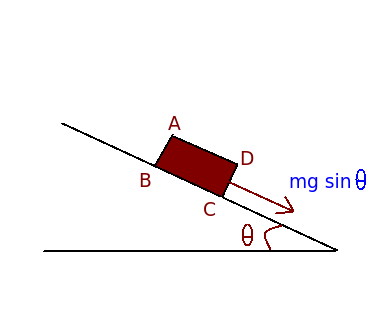# Acceleration on inclined plane less than acceleration due to gravity

Q.  A block sliding down an inclined plane has acceleration _________ acceleration due to gravity.
- Published on 21 Sep 15

a. less than
b. greater than
c. same as
d. none of the above

#### Discussion

• Sravanthi   -Posted on 15 Dec 15According to Newton's 2nd law, rate of change of linear momentum is directly proportional to impressed force.

Force = mass x acceleration

Force acting in downward direction = mg sin θ

Therefore, mg sin θ = ma

Acceleration of sliding block = acceleration due to gravity x sin θ

Hence, it is proved that acceleration of block ABCD sliding down an inclined plane is less than acceleration due to gravity.

## ➨ Post your comment / Share knowledgeEnter the code shown above:

(Note: If you cannot read the numbers in the above image, reload the page to generate a new one.)